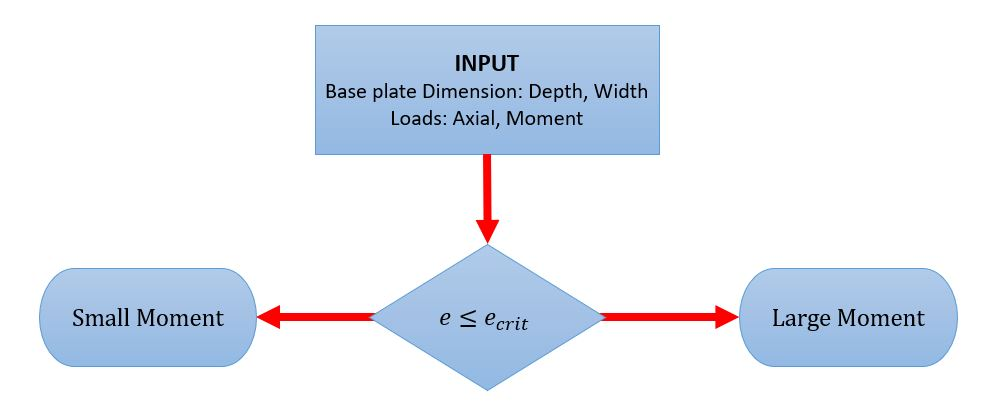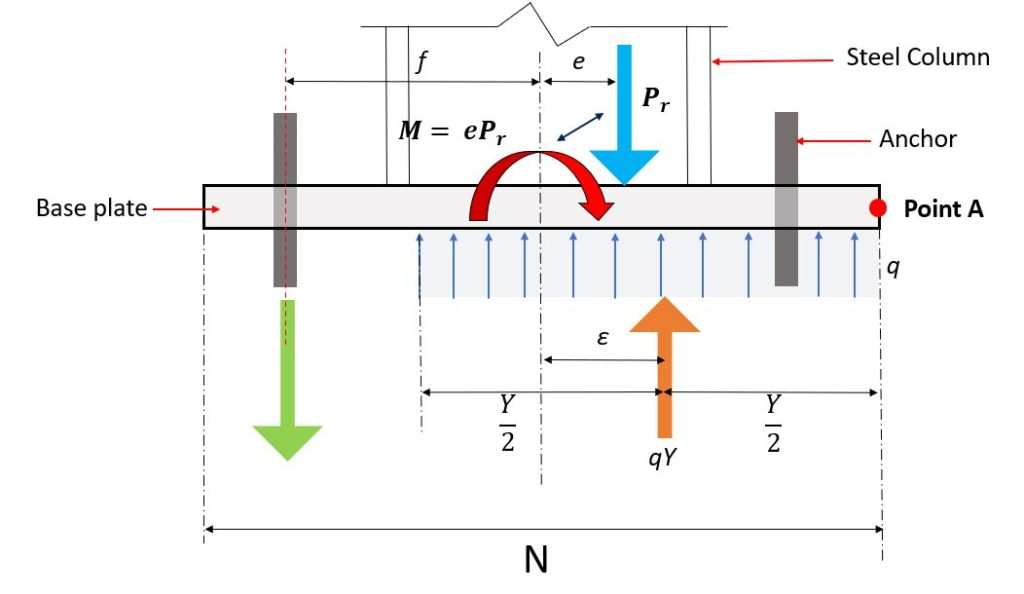SkyCiv文档

1. SkyCiv连接设计
2. 钢底板 (钢底板)

# 钢底板 (钢底板)

### 小力矩钢底板的设计

SkyCiv 钢底板 采用与静态平衡方程一致的设计方法修改的设计方法方法和 Doyle 和 Fisher 修改的 LRFD 方法 (2005) 并考虑两种带力矩的底板:

1. 小时刻 – 外部载荷仅由轴承抵抗
2. 大力矩 – 外部荷载由锚路和支座共同抵抗.

### 免费试用 SkyCiv 底板计算器:1. 在 SkyCiv 输入轴向载荷和力矩 钢底板.
2. 在 SkyCiv 输入底板尺寸 钢底板

$$一个_{1} = N times B$$

3. 计算等效偏心率的模块,

$$e = frac{中号}{ñ}$$

$$e_{暴击} = frac{ñ}{2} – \压裂{ P_{[R} }{ 2 \次q_{最高} }$$

4. 计算轴承长度的模块, 和.

5. 该模块计算所需的最小底板厚度 $$tp_{请求}$$.

6. 计算锚杆尺寸的模块 (如果需要).

SkyCiv 钢底板 考虑如图所示的力图 2.$$q = f_{p} \倍B$$

$$F_{p}$$ = 板和混凝土之间的承载应力
$$乙$$ = 底板宽度

$$ε = frac{ñ}{2} – \压裂{和}{2}$$

$$Y_{分} = frac{ P_{[R} }{ q_{最高} }$$

$$ε_{最高} = frac{ñ}{2} – \压裂{ Y_{分} }{ 2 } = frac{ñ}{2} – \压裂{ P_{ü} }{ 2 \次q_{最高} }$$

$$e = frac{中号}{ñ}$$## 参考资料

1. 钢结构设计指南底板和锚杆设计第二版. 美国钢结构学会, 2006.